# String to lowercase JavaScript (A detailed look)

In this tutorial, let’s look at different ways of converting a given string to lowercase.

There are multiple ways of doing the same, one of the easiest is to convert every character into lowercase, regardless of where it is. This means we’ll be converting the first letter in a sentence to lowercase as well. Let’s look at ways to do that first.

## Using the toLowerCase() method

One of the easiest ways to convert a string to lowercase is by using Javascript’s toLowerCase() method.
It’s quite simple. The syntax is as follows:

`string.toLowerCase()`

The above syntax will produce a lowercase version of the given string.

Let’s look at an example of how to do implement this function.

I’ll declare a variable “a” and assign it a string “Hello World”.

`var a = "Hello World";`

Then, I’ll use the toLowerCase() method to display the lowercase version of the string.

`document.write(a.toLowerCase());`

The above lines of code will produce the following output: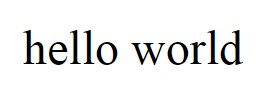Notice how the “H” in “Hello” and “W” in “World” are now in lowercase. So, this function goes through the entire string and makes every letter lowercase.

Let’s look at another example where every letter is uppercase.

`var a = "CAPITAL LETTERS";`

If I display this string with the toLowerCase() function, I’ll get the following output.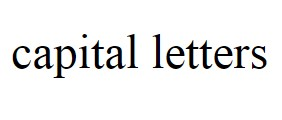Every letter was converted to lower case.

What if a string has no uppercase letters at all? What would the output be in that case? Let’s see.

`var a = "small letters";`

If I apply this new string to the function, I’ll get the following output: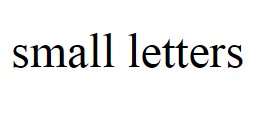There was no change in the string at all because this function only works on uppercase letters.

So, that’s how you can use Javascript’s built-in toLowerCase() method to convert a given string completely to lowercase.

Before we move on to the next section, I want to point out a few things to you.

Did you notice the capital letters in the toLowerCase() method? Both “L” and “C” are in uppercase. Javascript is a case sensitive language, even more so for its predefined function.

So, while using this function, make sure that you’re using uppercase for the required letters. If you don’t, the function won’t work.

I also want to point out the fact that this function does not change the original value of the string.

If you noticed in the previous example, the value of the string “a” will remain the same, even though we applied the toLowerCase() function on it. Let me prove it to you.

```var a = "Hello World";
document.write(a.toLowerCase() + "<br/>");
document.write(a);```

So, we’ve displayed 2 strings, the lowercase version, and then the original version, after the toLowerCase() method was applied on it. Let’s look at the output we get: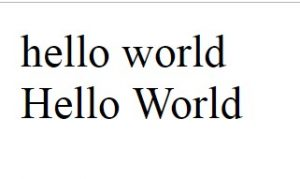As you can see, the original string remains the same.

But you can assign the new lowercase string to another variable, if that’s what you want to do.

```var a = "Hello World";
var b = a.toLowerCase();
document.write(b);```

Now, the string “b” will hold the lowercase version of the string “a”.

If you want to affect the original value of the string, then you’ll have to assign the lowercase version back to the original string.

```var a = "Hello World";
a = a.toLowerCase();
document.write(a);```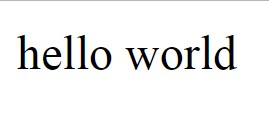Now, the string “a” has changed.

## The toLocaleLowerCase() Method

You can also use the toLocaleLowerCase() method to convert an entire string to lowercase, that is, to convert all of its characters to lowercase.

This is also a predefined Javascript method, and it works similar to the toLowerCase() method, the only difference being this method converts a given string to lowercase based on the user’s current locale; that is, the locale of the browser on which the program is run.

It looks at the language setting of the browser and spits out an output based on that.

This shouldn’t affect your output at all, except for in certain languages like Turkish. This is because these languages conflict with the regular Unicode case mappings, so you might get differing outputs in these cases.

Similar to the toLowerCase() method, the toLocaleLowerCase() method does not change the original value of the string as well. If you want to change the original value, you’ll have to apply the lowercase version of the string back to the original string/variable like we saw in the previous example.

Let’s look at an example of how this function works:

```var a = "CAPital LETTErs";
document.write(a.toLocaleLowerCase());```

The output we get is as follows:As you can see, I’m getting the same output as the toLowerCase() method because my browser’s language setting is English.

## Manual way of achieving the same

As I always say, it’s always good to learn the manual ways of solving problems to understand the problem and the programming language better.

So, let’s look at the manual way of converting a string to lowercase, that is, without using either the toLowerCase() or the toLocaleLowercase() methods.

The algorithm for the function we’ll be creating for this purpose is as follows:

1. Create an empty string. This string will hold the lowercase string and we’ll be returning this at the end of the function.

2. We’ll run a for loop through the string.

3. Then, we’ll calculate the ASCII code of every character in the string, for every run of the loop.

4. If the ASCII code is >= 65 and <=90, which means it falls anywhere between A to Z (capital letters), then we’ll add 32 to the ASCII code, retrieve the relevant character for the new ASCII code and append it to the new string we created. If you look at ASCII codes, the ASCII code of “A” is 65, but for “a”, it’s 97, which is 65 + 32. So, by adding 32 to ASCII codes that correspond to capital letters, we get their respective lower letter versions.

5. If the letter is a small letter or a special character, that is, its ASCII code does not fall between 65 and 90, then we affix the character as is to the new string.

6. Finally, return the string.

That’s it. It’s a very simple algorithm. Let’s implement it in code now. I’ll explain the code line by line first, and then print out the entire code for your convenience.

Let’s first declare the string and receive the string as the argument “str”.

`function stringLower(str) {`

Then, let’s create the new string and assign it an empty string to start with.

`var nStr = "";`

Then, let’s create a for loop that’ll loop through the entire string.

`for(var i=0; i<str.length; i++) {`

For every iteration of the loop, we will calculate the ASCII character of the particular character the loop is at. This is calculated by using the “i” value of the string “str”.

`var code = str.charCodeAt(i);`

So, this above code basically asks for the ASCII code of the ith character of the string “str”.

For example, if the string is “cat”, then str.charCodeAt(0) will give the ASCII code of the character “c”, since that’s the first character.

In strings, the first character is always referenced with the number 0, so if you want to access the character “c” of the above string, then you should access it like this: str.

To access “a”, use str and so on.

So, now that we’ve calculated the ASCII code of the ith character of the string, we can check if it’s a capital letter or not.

`if(code >= 65 && code <= 90) {`

As you know, the ASCII code of “A” is 65 and that of “Z” is 90, and the other capital letters’ ASCII codes are between 65 and 90, so if the retrieved ASCII code, that is, the one in the variable “code” is between 65 and 90, we can conclude that it’s an uppercase character.

Let’s convert it to a lowercase character now.

`nStr += String.fromCharCode(code+32);`

In the above line of code, we are adding 32 to the “code” value so we get the lowercase ASCII equivalent of the uppercase character.

Then, we’re using Javascript’s built-in function String.fromCharCode() to retrieve the character equivalent of the given ASCII code.

Once that’s retrieved, we are appending that character to the “nStr” string.

Did you notice how we used “+=” to do the concatenation? That’s because we need to add the new character to the end of the string, while still retaining the characters that were previous added to the string in previous loop iterations.

If the ASCII code value does not fall between 65 to 90, then the character is either a lowercase character, number or a special character, so we can affix it to “nStr” as it is.

```else {
nStr += str[i];
}```

Let’s let the for loop run for the entirety of the string’s length, and once it’s finished running, return the new string nStr back to the from where the function call originated.

`return nStr;`

That’s it. This is how you can convert a string to lowercase without using any of the pre-defined Javascript functions used for this purpose.

Let me show you the entire code so you can replicate it.

```function stringLower(str) {
var nStr = "";
for(var i=0; i<str.length; i++) {
var code = str.charCodeAt(i);
if(code >= 65 && code <= 90) {
nStr += String.fromCharCode(code+32);
}
else {
nStr += str[i];
}
}
return nStr;
}
var a = "MANUAL function";
document.write(stringLower(a));```

If we run this program, this is the output we’ll get: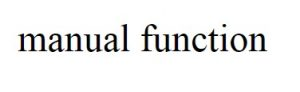## Converting everything except the first letter of the string to lowercase

Now that we know how to convert all characters of a given string to lowercase, let’s go a step further and try to do the same for every character, except the first character.

The premise is that, a sentence usually starts with a capital letter, so we are to leave it as it is.

I’ll just be making some small changes to make this happen.

`var nStr = str;`

When declaring the string “nStr”, I’ll initialize it with the first character of the string “str”.

Then, I’ll run the for loop from i=1, and not i=0, so the loop only goes through the second character of the string “str” to the end of the string and omit the first character. So, if the first character is a capital letter, then it’ll remain a capital letter, and it was already assigned to the new string.

`for(var i=1; i<str.length; i++) {`

That’s it. These are the only 2 changes we’ll be making. You can edit these 2 lines of code in the program I’ve printed above, and if you run the same program, this is the output you’d get: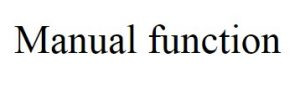Notice how the first character “M” is still a capital letter, but the rest were converted to lowercase? We’ve achieved our objective.

These are the various ways in which you can change a given string to lowercase.